Contents

Idea

In the context of string theory the NS5-brane is a certain extended physical objects – a brane – that appears in/is predicted by the theory.

There are different incarnations of this object:

For instance the effective background QFT of the type II stringtype II supergravity – admits solutions to its generalized Einstein equations which describe higher dimensional analogs of charged black holes in ordinary gravity. Among them is a 5+1-dimensional “black brane” which is magnetically charged under the Kalb-Ramond field. Since the KR field and the field of gravity constituting this solution of type II supergravity have as quanta the worldsheet excitations of the spinning string sigma-model that sit in what is called the Neveu-Schwarz sector? one calls this the NS5-brane.

This is to distinguish it from the D5-brane which is instead charged under the RR-field whose quanta come from the Ramond-Ramond sector? of the superstring.

There are other incarnations of the NS 5-brane:

by the general logic of higher electromagnetism the (1+1)-dimensional string has under electric-magnetic duality a magnetic dual . By dimension counting this is a 5-brane. If we think of the string this way as the structure that supports the sigma-model that defines perturbative string theory, we also call it the F1-brane (the fundamental 1-brane). In this sense the the corresponding magnetic dual is the F5-brane – the fundamental fivebrane.

One can understand the NS5-“black brane” solution to type II supergravity as being the solitonic incarnation of the fundamental 5-brane in much the same way as an ordinary black hole in ordinary gravity is a solitonic incarnation of the fundamental particle: as the particle, the black hole it is characterized just by mass, charge and angular momentum.

Similarly, the “black” NS5-brane is characterizes by mass, B-field charge and angular momentum.

Properties

As a black brane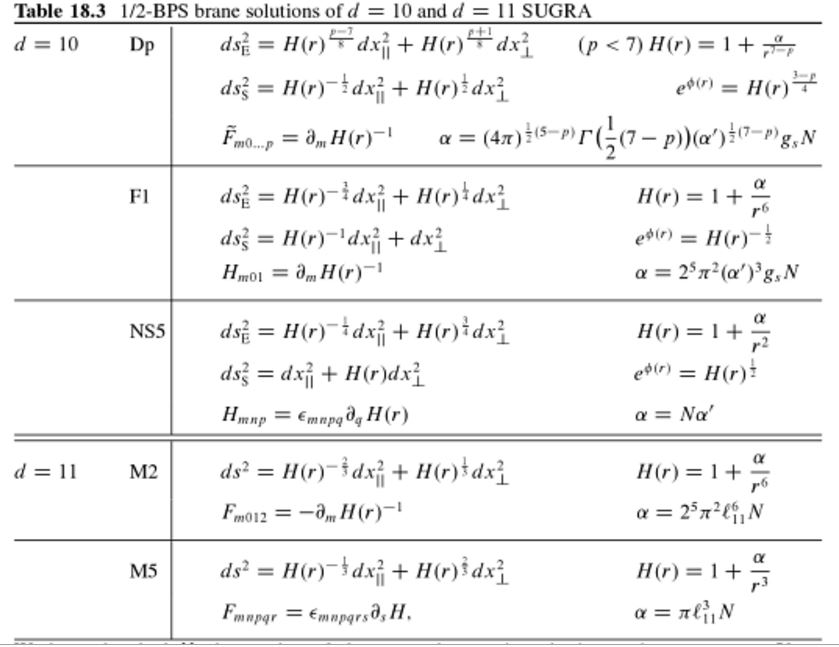(table taken from Blumenhagen-Lüst-Theisen 13, Chapter 18.5)

Relation to little string theory

By the brane scan, on the worldvolume of an NS5-brane propagates a superstring. This is called the little string, see there for more.

D-branes ending on NS5-branes

This ought to be this way if S-duality and T-duality work as expected, since:

$\array{ \text{F1 on D5} &\overset{\text{S}}{\leftrightarrow}& \text{D1 on NS5} \\ && \updownarrow\mathrlap{T} \\ && \text{D2 on NS5} \\ && \updownarrow\mathrlap{T} \\ && \text{D3 on NS5} &\overset{\text{S}}{\leftrightarrow}& \text{D3 on D5} \\ && \updownarrow\mathrlap{T} \\ && \vdots }$

This leads to what is called geometric engineering of quantum field theory on the worldvolume of these branes (following Hanany-Witten 97, review includes Fazzi 17).

Open D$p$-brane theories on the NS5

Combining the above two items, the corresponding worldvolume theories of the NS5-branes (see also at little string theory) in the presence of light D$p$-branes ending on/inside them are also referred to as “open D$p$-brane theories” or “OD$p$ theories” (Gopakumar-Minwalla-Seiberg-Strominger 00, section 6, Harmark 00, section 4.2, Lu 06, section 6)

D4-branes ending on NS5-branes

see Witten 97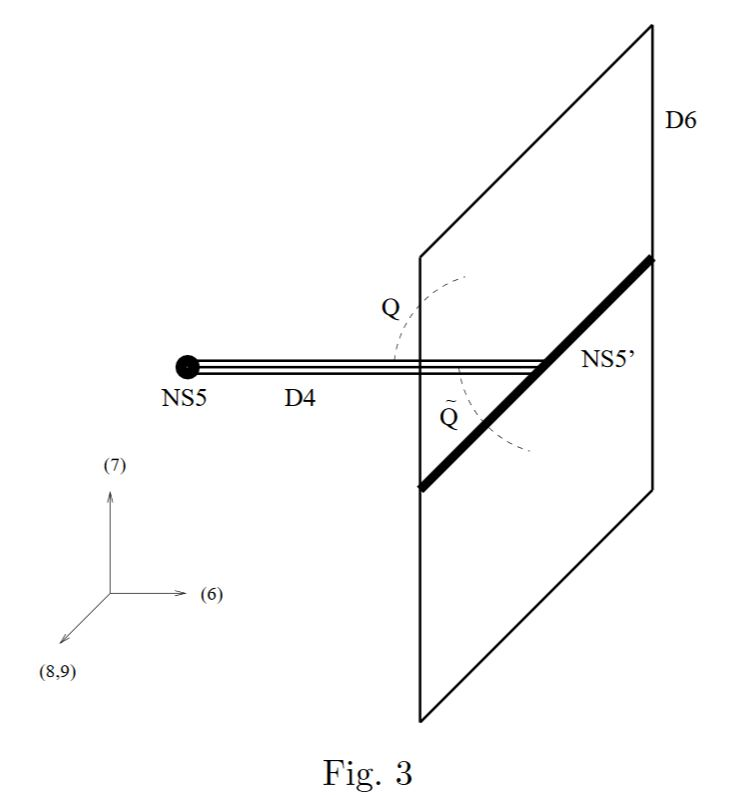graphics grabbed from EGKRS 00

D6-branes ending on NS5-branes

Consider a black NS5-brane with near horizon geometry $\underset{\sim AdS_7}{\underbrace{ \mathbb{R}^{5,1} \times \mathbb{R}_{\phi} }} \times S^3$ (EGKRS 00, p. 8):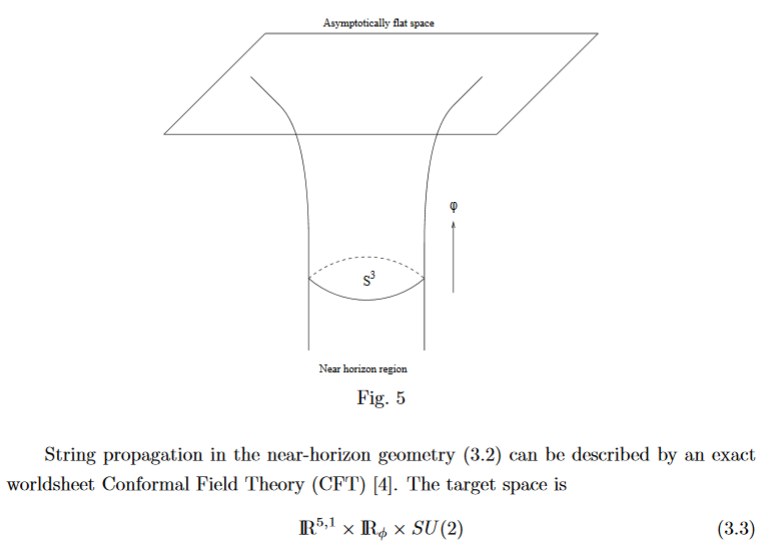The 3-sphere factor $S^3$ is the unit sphere around the black NS5-brane worldvolume $\mathbb{R}^{5,1}$, and $\mathbb{R}_{\phi}$ parameterizes the radial distance from it.

Placing a D6-brane at one point of the $S^3$-factor (EGKRS 00, p. 20)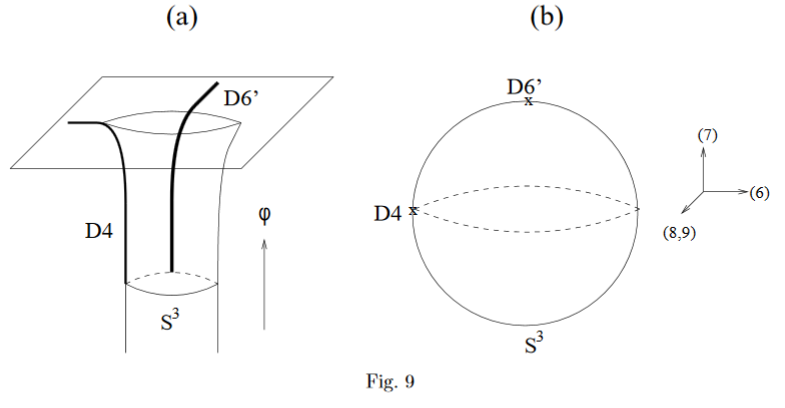means to take its worldvolume to be the factor $\mathbb{R}^{5,1} \times \mathbb{R}_\phi$, hence extending to one side of the NS5-brane (EGKRS 00, p. 7):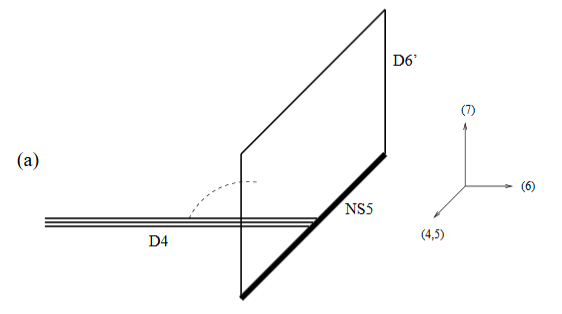Placing another D6-brane at the corresponding antipodal point means to have it extend also to the other side (EGKRS 00, p. 5):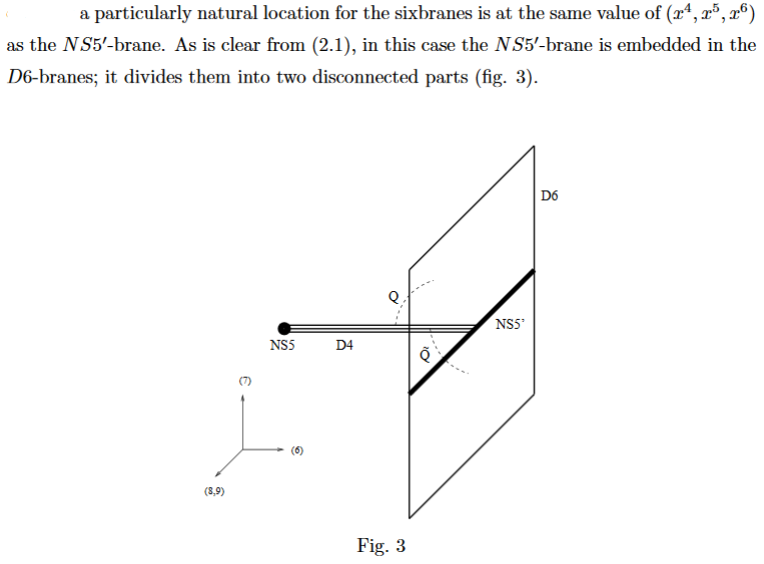or else to have embedded the black NS5-brane into a single D6-brane.

Special properties D6-branes ending on NS5-branes were highlighted in (Brodie-Hanany 97, section 2.4): the worldvolume theory becomes chiral.

The M-theory-lift of this situation should be the 4-spherical orbifold near horizon geometry of an M5-brane (MFF 12, section 8.3)

$\underset{\sim AdS_7}{\underbrace{\mathbb{R}^{5,1} \times \mathbb{R}_\phi}} \times S^4/G_{ADE}$

where $G_{ADE} \subset SU(2)$ is a finite subgroup of SU(2) (hence in the ADE classification), acting via the identification $S^4 \simeq S(\mathbb{H} \oplus \mathbb{R})$ (see at 4-sphere the section SU(2)-action). For non-trivial $G_{ADE}$, this action has precisely two fixed points $S^0 \hookrightarrow S^4 \to S^3$. Hence $\mathbb{R}^{5,1} \times \mathbb{R}_\phi \times S^0$ must be the worldvolume of two KK-monopoles of 11d supergravity, which is the M-theory lift of the two D6-branes. While the M-theory lift of the NS5-brane is the M5-brane with worldvolume $\mathbb{R}^{5,1}$. See also Fazzi 17, p. 38:

The NS5-D6 Hanany–Witten setup engineering six-dimensional $(1,0)$ theories is equivalent to M5-branes at an $A_k$ singularity in eleven dimensions.

Next, this construction may be repeated, having the D6-branes end on different NS5-branes, hence “suspended between NS5-branes” (graphics from Fazzi 17, p. 33):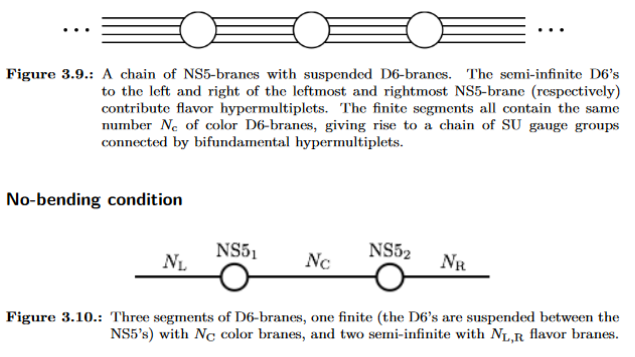D6 branes intersecting D8-branes over NS5-branes

…and on the other end, the D6-branes may end on D8-branes over an NS5-brane (Janssen-Meessen-Ortin 99, Section 3) yielding D6-D8 brane intersections: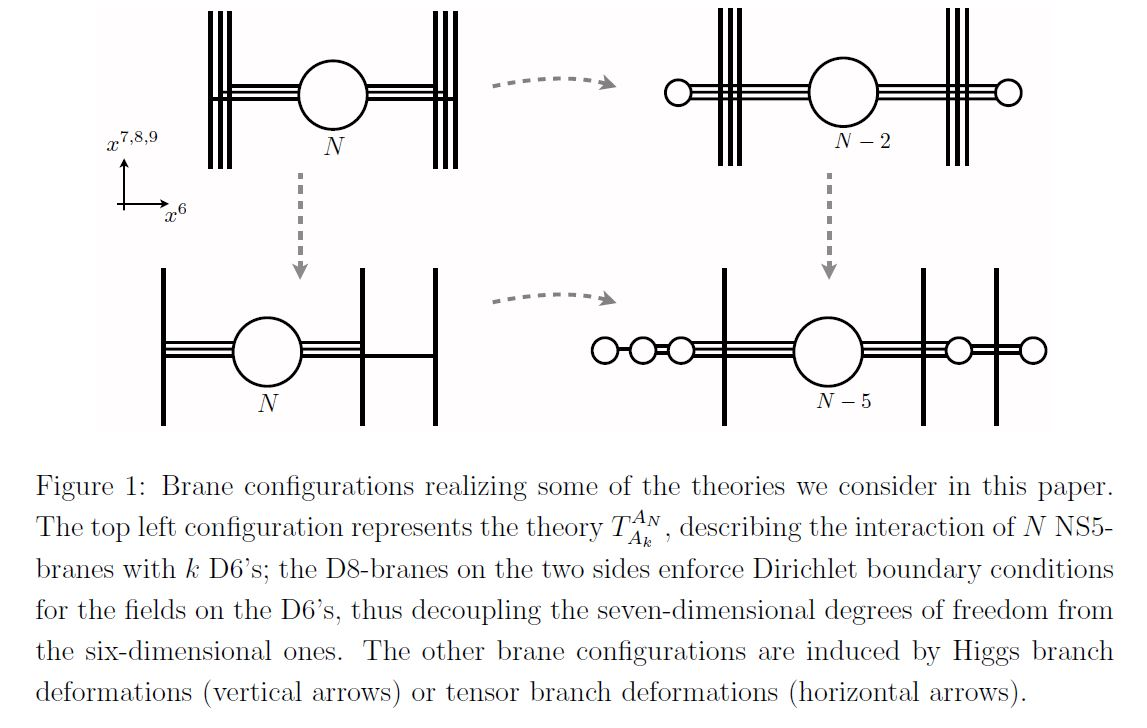graphics grabbed from Gaiotto-Tomasiello 14

Subject to the s-rule: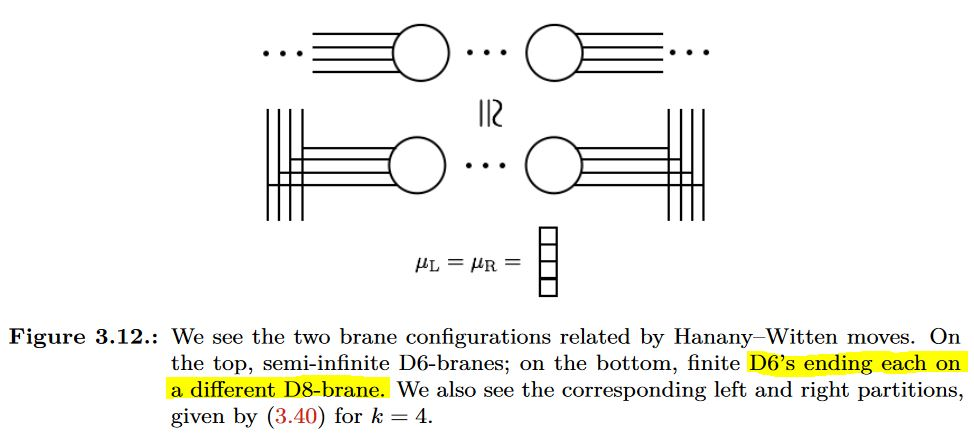graphics grabbed from Fazzi 17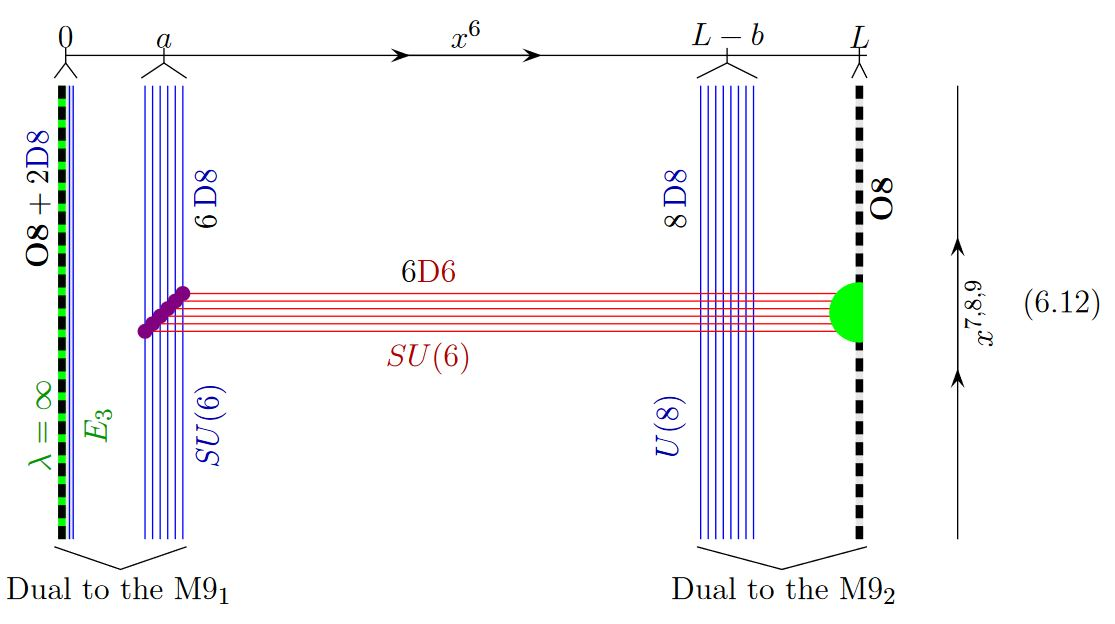graphics grabbed from GKSTY 02

fuzzy funnel$\,$noncommutative geometry: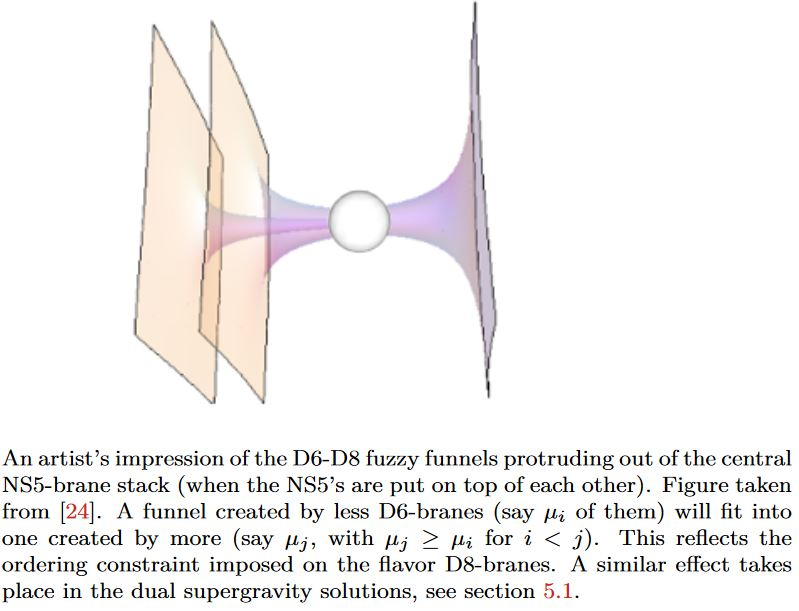graphics grabbed from Fazzi 17, Fig. 3.14, taken in turn from Gaiotto-Tomassiello 14, Figure 5

Generally Dp-D(p+2)-brane intersections geometrically engineer Yang-Mills monopoles in the worldvolume of the higher dimensional D$(p+2)$-branes.

Specifically for $p = 6$, i.e. for D6-D8-brane intersections, this fits with the Witten-Sakai-Sugimoto model geometrically engineering quantum chromodynamics, and then gives a geometric engineering of the Yang-Mills monopoles in actual QCD (HLPY 08, p. 16).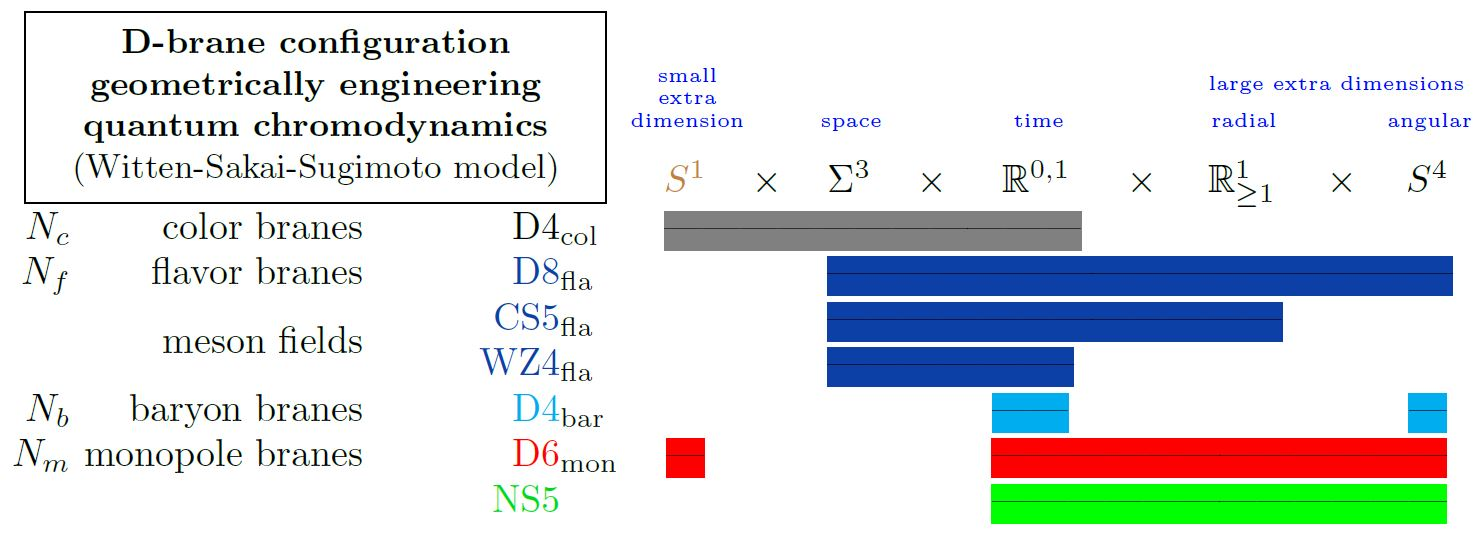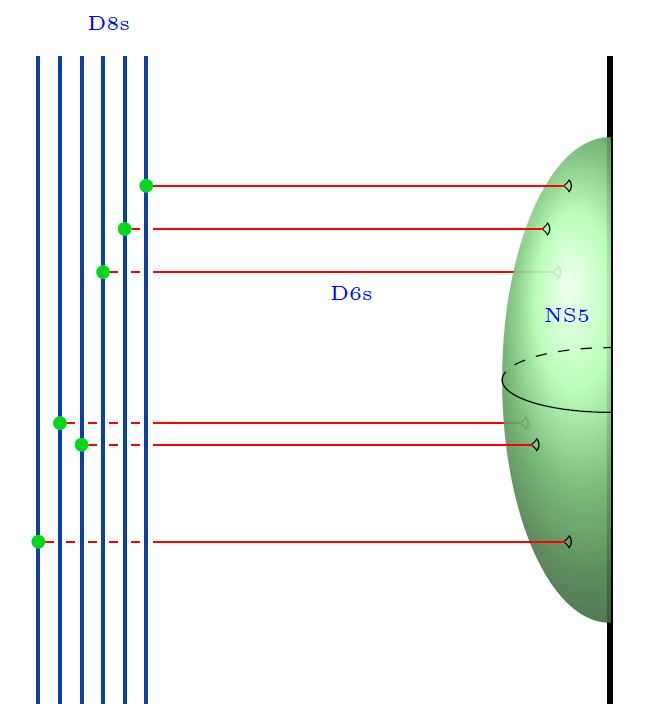Here we are showing

1. the color D4-branes;

2. the flavor D8-branes;

with

1. the 5d Chern-Simons theory on their worldvolume

2. the corresponding 4d WZW model on the boundary

both exhibiting the meson fields

3. (see at WSS – Baryons)

4. (see at D6-D8-brane bound state)

5. the NS5-branes.

graphics grabbed from Sati-Schreiber 20

NS5 half-branes

By the discussion above, a black D6-brane may end on a black NS5-brane, and in fact a priori each brane NS5-brane has to be the junction of two black D6-branes.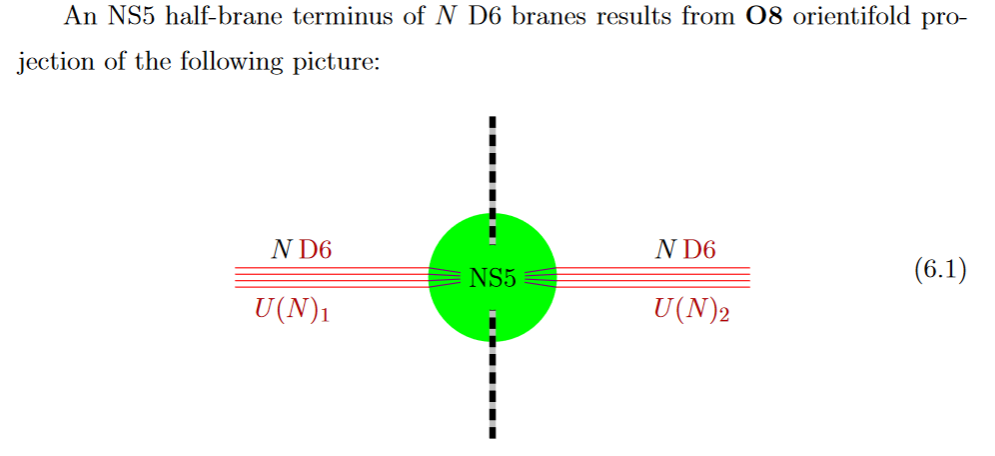from GKSTY 02

If in addition the black NS5-brane sits at an O8-plane, hence at the orientifold fixed point-locus, then in the ordinary $\mathbb{Z}/2$-quotient it appears as a “half-brane” with only one copy of D6-branes ending on it: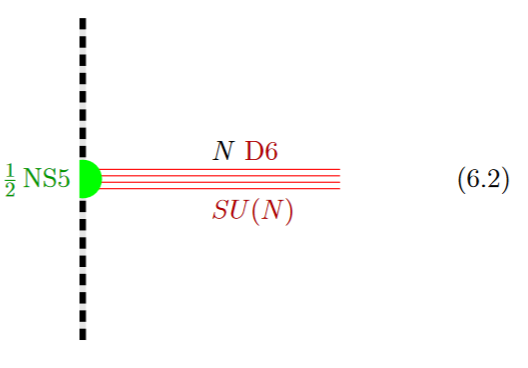from GKSTY 02

(In Hanany-Zaffaroni 99 this is interpreted in terms of the 't Hooft-Polyakov monopole.)

The lift to M-theory of this situation is an M5-brane intersecting an M9-brane (see at MO5-plane and at heterotic M-theory on ADE-orbifolds):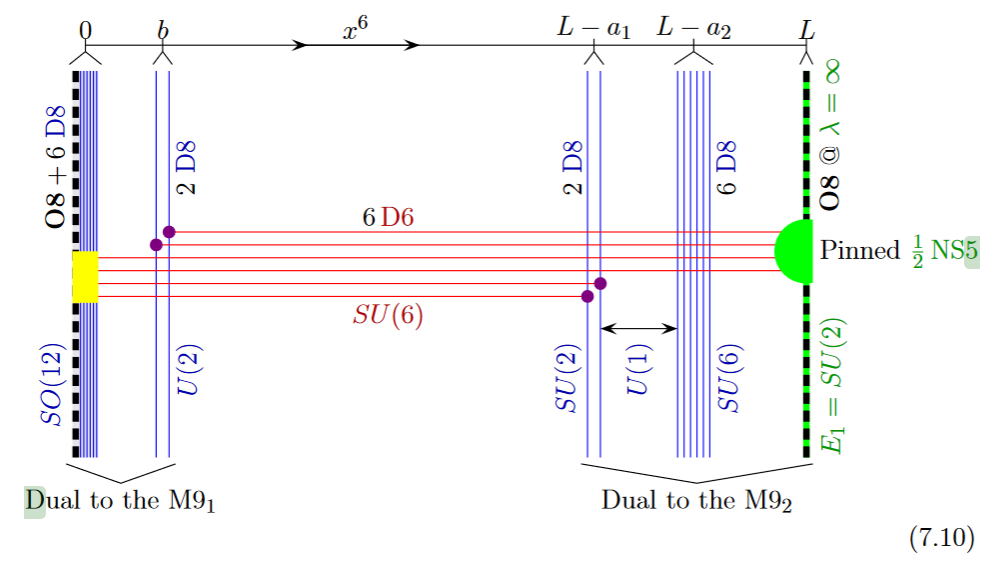from GKSTY 02

Alternatively the O8-plane may intersect the black D6-branes away from the black NS5-brane: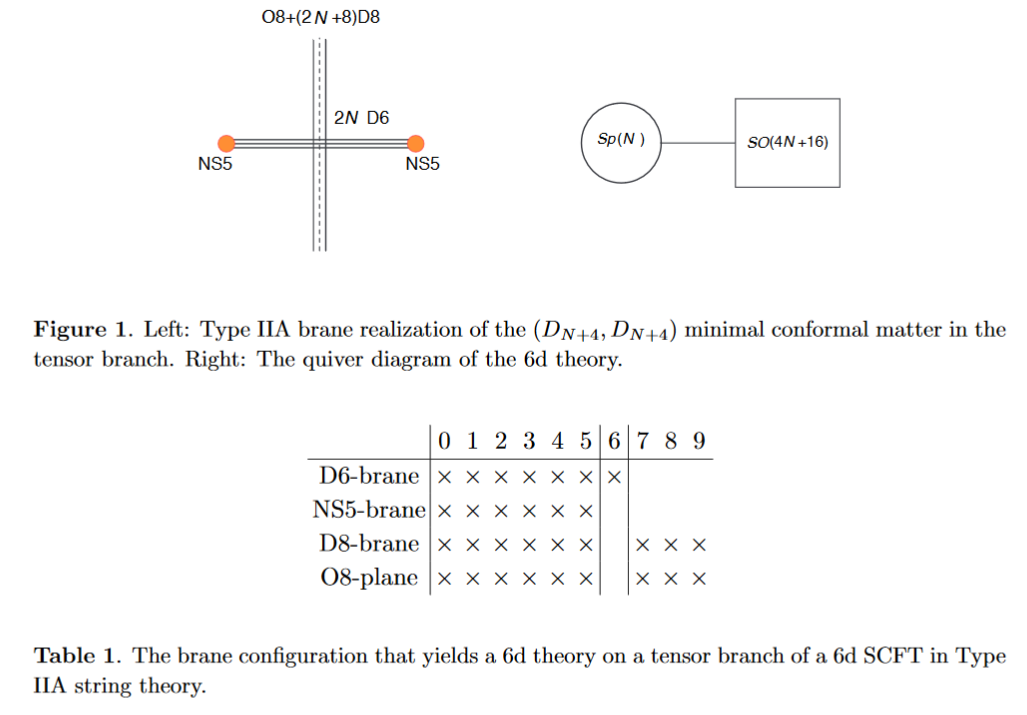from HKLY 15

In general, some of the NS5 sit away from the O8-plane, while some sit on top of it: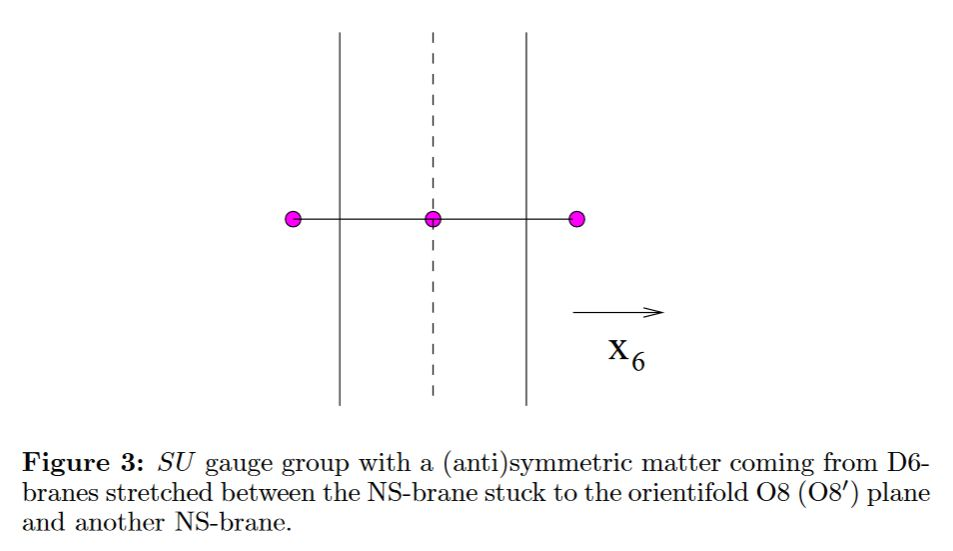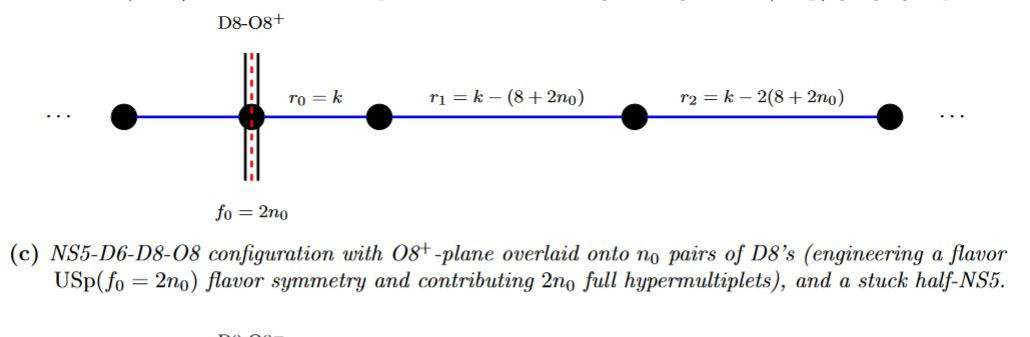graphics grabbed from Apruzzi-Fazzi 17, p. 18

The lift to M-theory under duality between M-theory and heterotic string theory is the half M5-brane.

See also at intersecting D-brane models the section Intersection of D6s with O8s.

Webs

corresponding brane webs: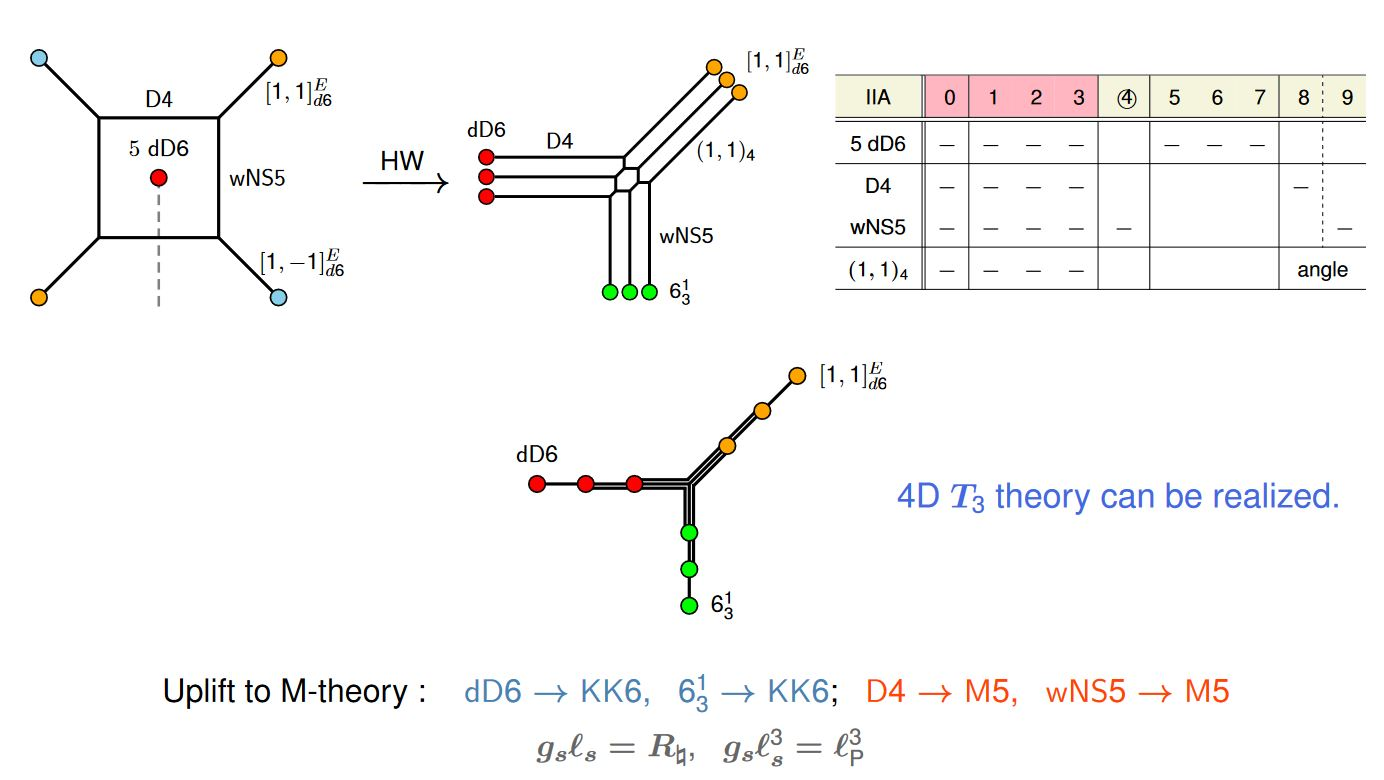graphics grabbed from (Kimura 16 pdf)

NS5/D4/D2 bound states

bound states of NS5-branes with D4-branes and D2-branes: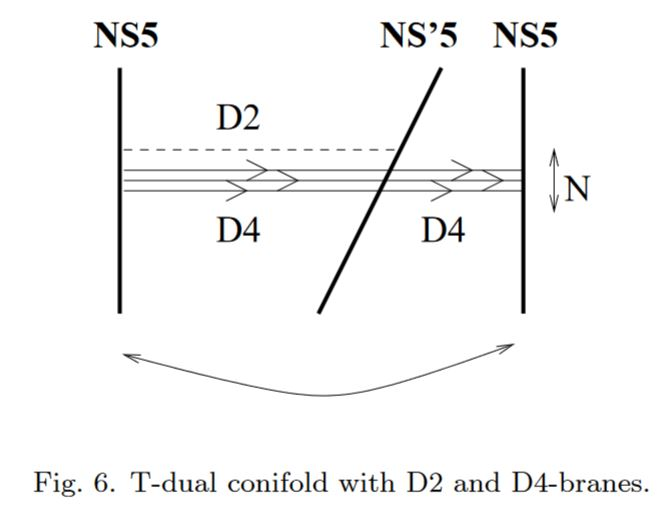graphics grabbed from Mukhi-Suryanarayana 00

Notice that this includes configurations with the D4-branes and D2-branes contained strictly inside the NS5: Mitra-Roy 00, section 4

Review includes Camino 02, section 4.5

For the lift to M-theory see at M2-M5 brane bound state

Relation to Khovanov homology

Khovanov homology has long been expected to appear as the observables in a 4-dimensional TQFT in higher analogy of how the Jones polynomial arises as an observable in 3-dimensional Chern-Simons theory. For instance for $\Sigma : K \to K'$ a cobordism between two knots there is a natural morphism

$\Phi_\Sigma : \mathcal{K}(K) \to \mathcal{K}(K')$

between the Khovanov homologies associated to the two knots.

In (Witten11) it is argued, following indications in (GukovSchwarzVafa) that this 4d TQFT is related to the worldvolume theory of the image in type IIA of D3-branes ending on NS5-branes in type IIB after one S-duality and one T-duality operation:

$(D3 - NS4) \stackrel{S}{\mapsto} (D3 - D5) \stackrel{T}{\mapsto} (D4-D6) \,.$

Earlier indication for this had come from the observation that Chern-Simons theory is the effective background theory for the A-model 2d TCFT (see TCFT – Worldsheet and effective background theories for details).

Notice that after the above T-duality operation the $(D4-D6)$-system wraps the $S^1$ (circle) along which the T-duality takes place.

Lifting that configuration to 11-dimensional supergravity gives M5-branes (the erstwhile D4-branes) on Taub-NUT ($\times S^1$). The M5-branes wrap the circle-fiber of Taub-NUT, which shrinks to zero size at the origin (the location of the erstwhile D6, which is where the D4s “end”). The low-energy theory, on a stack of M5-branes, is the 6d (2,0)-susy QFT.

Table of branes appearing in supergravity/string theory (for classification see at brane scan).

branein supergravitycharged under gauge fieldhas worldvolume theory
black branesupergravityhigher gauge fieldSCFT
D-branetype IIRR-fieldsuper Yang-Mills theory
$(D = 2n)$type IIA$\,$$\,$
D(-2)-brane$\,$$\,$
D0-brane$\,$$\,$BFSS matrix model
D2-brane$\,$$\,$$\,$
D4-brane$\,$$\,$D=5 super Yang-Mills theory with Khovanov homology observables
D6-brane$\,$$\,$D=7 super Yang-Mills theory
D8-brane$\,$$\,$
$(D = 2n+1)$type IIB$\,$$\,$
D(-1)-brane$\,$$\,$$\,$
D1-brane$\,$$\,$2d CFT with BH entropy
D3-brane$\,$$\,$N=4 D=4 super Yang-Mills theory
D5-brane$\,$$\,$$\,$
D7-brane$\,$$\,$$\,$
D9-brane$\,$$\,$$\,$
(p,q)-string$\,$$\,$$\,$
(D25-brane)(bosonic string theory)
NS-branetype I, II, heteroticcircle n-connection$\,$
string$\,$B2-field2d SCFT
NS5-brane$\,$B6-fieldlittle string theory
D-brane for topological string$\,$
A-brane$\,$
B-brane$\,$
M-brane11D SuGra/M-theorycircle n-connection$\,$
M2-brane$\,$C3-fieldABJM theory, BLG model
M5-brane$\,$C6-field6d (2,0)-superconformal QFT
M9-brane/O9-planeheterotic string theory
M-wave
topological M2-branetopological M-theoryC3-field on G2-manifold
topological M5-brane$\,$C6-field on G2-manifold
S-brane
SM2-brane,
membrane instanton
M5-brane instanton
D3-brane instanton
solitons on M5-brane6d (2,0)-superconformal QFT
self-dual stringself-dual B-field
3-brane in 6d

\linebreaK

As a black brane

The 5-brane in heterotic string theory was found as a black brane in

The intersection laws with black D-branes are discussed in

Review includes

The M-theory-lift of the black NS5-brane embedded into a D6-brane should be the configuration from section 8.3 of

As a Green-Schwarz sigma-model

The Green-Schwarz action functionals for the NS5-brane:

as a higher WZW model:

Under dualities

Discussion of the effect of T-duality on NS5-branes includes

Relation to the M5-brane

Most of the following references are more on the M5-brane.

The fact that the worldvolume theory of the M5-brane should support fields that are self-dual connections on a 2-bundle ($\sim$ a gerbe) is discussed in

as well as sections 3 and 4 of

A review of some aspects is in

The relation to Khovanov homology is discussed in

• Greg Moore, On the role of six‐dimensional $(2,0)$-theories in recent developments in

Physical Mathematics_ , talk at Strings2011 (pdf slides)

The above discussion makes use of some blog comments (notably by Jacques Distler) appearing at

Intersection with O8/D8

Intersection of black NS5-branes with O8-planes/black D8-branes is discussed in

On the orientifold T-dual of half M5-branes:

For more see at M-theory on S1/G_HW times H/G_ADE.

NS5/D4/D2 bound states

bound states of NS5-branes with D4-branes and D2-branes (see also at M2-branes – Dyonic membranes)

• Indranil Mitra, Shibaji Roy, (NS5,Dp) and (NS5,D(p+2),Dp) bound states of type IIB and type IIA string theories, JHEP 0102:026, 2001 (arXiv:hep-th/0011236)

• Mohsen Alishahiha, Yaron Oz, Supergravity and “New” Six-Dimensional Gauge Theories, Phys.Lett.B495:418-426, 2000 (arXiv:hep-th/0008172)

• Indranil Mitra, Shibaji Roy, (NS5,D5,D3) bound state, OD3, OD5 limits and SL(2,Z) duality, Phys.Rev. D65 (2002) 106001 (arXiv:hep-th/0107127)

• Sunil Mukhi, Nemani V. Suryanarayana, Stable Non-BPS States and Their Holographic Duals, Int. J. Mod. Phys. A16 (2001) 966-975 (arXiv:hep-th/0011185)

• J. M. Camino, section 4.5 of Worldvolume Dynamics of Branes (arXiv:hep-th/0210249)

• Qiang Jia, J. X. Lu, Shibaji Roy, On 1/4 BPS ((F, D1), (NS5, D5)) bound states of type IIB string theory, JHEP 08 (2017) 007 (arXiv:1704.01463)

• Giuseppe Dibitetto, Nicolò Petrim, 6d surface defects from massive type IIA, JHEP 01 (2018) 039 (arXiv:1707.06154)

• Nicolò Petri, Surface defects in massive IIA, talk at Recent Trends in String Theory and Related Topics 2018 (pdf)